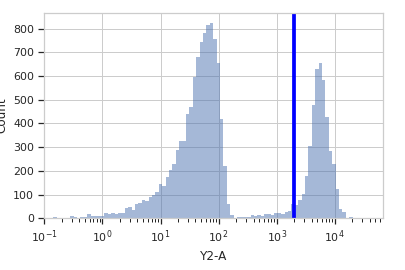# cytoflow.operations.threshold¶

class cytoflow.operations.threshold.ThresholdOp[source]

Apply a threshold gate to a cytometry experiment.

name

The operation name. Used to name the new column in the experiment that’s created by apply()

Type: Str
channel

The name of the channel to apply the threshold on.

Type: Str
threshold

The value at which to threshold this channel.

Type: Float

Examples

Make a little data set.

>>> import cytoflow as flow
>>> import_op = flow.ImportOp()
>>> import_op.tubes = [flow.Tube(file = "Plate01/RFP_Well_A3.fcs",
...                              conditions = {'Dox' : 10.0}),
...                    flow.Tube(file = "Plate01/CFP_Well_A4.fcs",
...                              conditions = {'Dox' : 1.0})]
>>> import_op.conditions = {'Dox' : 'float'}
>>> ex = import_op.apply()


Create and parameterize the operation.

>>> thresh_op = flow.ThresholdOp(name = 'Threshold',
...                              channel = 'Y2-A',
...                              threshold = 2000)


Plot a diagnostic view

>>> tv = thresh_op.default_view(scale = 'log')
>>> tv.plot(ex)Note

If you want to use the interactive default view in a Jupyter notebook, make sure you say %matplotlib notebook in the first cell (instead of %matplotlib inline or similar). Then call default_view() with interactive = True:

tv = thresh_op.default_view(scale = 'log',
interactive = True)
tv.plot(ex)


Apply the gate, and show the result

>>> ex2 = thresh_op.apply(ex)
>>> ex2.data.groupby('Threshold').size()
Threshold
False    15786
True      4214
dtype: int64

apply(experiment)[source]

Applies the threshold to an experiment.

Parameters: experiment (Experiment) – the experiment to which this operation is applied a new experiment, the same as the old experiment but with a new column of type bool with the same name as the operation name. The new condition is True if the event’s measurement in channel is greater than threshold; it is False otherwise. Experiment
default_view(**kwargs)[source]
class cytoflow.operations.threshold.ThresholdSelection[source]

Plots, and lets the user interact with, a threshold on the X axis.

TODO - beautify!

interactive

is this view interactive?

Type: Bool
channel

The channel this view is viewing. If you created the view using default_view(), this is already set.

Type: String
scale

The way to scale the x axes. If you created the view using default_view(), this may be already set.

Type: {‘linear’, ‘log’, ‘logicle’}
op

The IOperation that this view is associated with. If you created the view using default_view(), this is already set.

Type: Instance(IOperation)
xfacet, yfacet

Set to one of the conditions in the Experiment, and a new row or column of subplots will be added for every unique value of that condition.

Type: String
huefacet

Set to one of the conditions in the in the Experiment, and a new color will be added to the plot for every unique value of that condition.

Type: String
huescale

How should the color scale for huefacet be scaled?

Type: {‘linear’, ‘log’, ‘logicle’}

Notes

We inherit xfacet and yfacet from cytoflow.views.HistogramView, but they must both be unset!

Examples

In an Jupyter notebook with %matplotlib notebook

>>> t = flow.ThresholdOp(name = "Threshold",
...                      channel = "Y2-A")
>>> tv = t.default_view()
>>> tv.plot(ex2)
>>> tv.interactive = True
>>> # .... draw a threshold on the plot
>>> ex3 = thresh.apply(ex2)

plot(experiment, **kwargs)[source]

Plot the histogram and then plot the threshold on top of it.

Parameters: experiment (Experiment) – The Experiment to plot using this view. title (str) – Set the plot title xlabel, ylabel (str) – Set the X and Y axis labels huelabel (str) – Set the label for the hue facet (in the legend) legend (bool) – Plot a legend for the color or hue facet? Defaults to True. sharex, sharey (bool) – If there are multiple subplots, should they share axes? Defaults to True. row_order, col_order, hue_order (list) – Override the row/column/hue facet value order with the given list. If a value is not given in the ordering, it is not plotted. Defaults to a “natural ordering” of all the values. height (float) – The height of each row in inches. Default = 3.0 aspect (float) – The aspect ratio of each subplot. Default = 1.5 col_wrap (int) – If xfacet is set and yfacet is not set, you can “wrap” the subplots around so that they form a multi-row grid by setting col_wrap to the number of columns you want. sns_style ({“darkgrid”, “whitegrid”, “dark”, “white”, “ticks”}) – Which seaborn style to apply to the plot? Default is whitegrid. sns_context ({“paper”, “notebook”, “talk”, “poster”}) – Which seaborn context to use? Controls the scaling of plot elements such as tick labels and the legend. Default is talk. despine (Bool) – Remove the top and right axes from the plot? Default is True. min_quantile (float (>0.0 and <1.0, default = 0.001)) – Clip data that is less than this quantile. max_quantile (float (>0.0 and <1.0, default = 1.00)) – Clip data that is greater than this quantile. lim ((float, float)) – Set the range of the plot’s data axis. orientation ({‘vertical’, ‘horizontal’}) num_bins (int) – The number of bins to plot in the histogram. Clipped to [100, 1000] histtype ({‘stepfilled’, ‘step’, ‘bar’}) – The type of histogram to draw. stepfilled is the default, which is a line plot with a color filled under the curve. density (bool) – If True, re-scale the histogram to form a probability density function, so the area under the histogram is 1. linewidth (float) – The width of the histogram line (in points) linestyle ([‘-’ | ‘–’ | ‘-.’ | ‘:’ | “None”]) – The style of the line to plot alpha (float (default = 0.5)) – The alpha blending value, between 0 (transparent) and 1 (opaque).